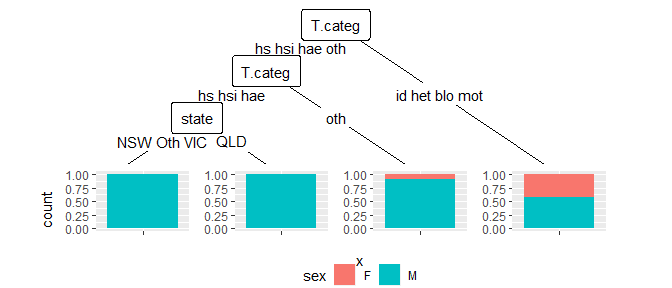# Parsing

Let’s take a closer look at how to use geom_edge_label().
In most cases you hopefully won’t have to worry much about this geom, since the defaults should produce satisfying results.
But if you do want to customize anything, it might get a bit tricky. Since splits of continuous variables contain intervals we want to be able to plot inequality signs. Using Unicode to do so, proved problematic among other things with some pdf engines. Therefore these signs are added as parsable text.
However, this opens the door to some other potential problems. To ensure correct behaviour as per default geom_edge_label() parses only these signs. Therefore the additional argument parse_all has been added which allows to parse the whole label if set to TRUE. First let’s once more recreate the WeatherPlay tree. But this time we are going to arbitrarily change the first level of outlook to “beta”

library(ggparty)
data("WeatherPlay", package = "partykit")
levels(WeatherPlay\$outlook) <- c("beta")
sp_o <- partysplit(1L, index = 1:3)
sp_h <- partysplit(3L, breaks = 75)
sp_w <- partysplit(4L, index = 1:2)
pn <- partynode(1L, split = sp_o, kids = list(
partynode(2L, split = sp_h, kids = list(
partynode(3L, info = "yes"),
partynode(4L, info = "no"))),
partynode(5L, info = "yes"),
partynode(6L, split = sp_w, kids = list(
partynode(7L, info = "yes"),
partynode(8L, info = "no")))))
py <- party(pn, WeatherPlay)

## Default Mapping

As per default geom_edge_label() maps label to plot_data’s breaks_label.
Plotting the tree in the usual way will lead to the following plot.

ggparty(py) +
geom_edge() +
geom_edge_label() +
geom_node_splitvar() +
geom_node_info()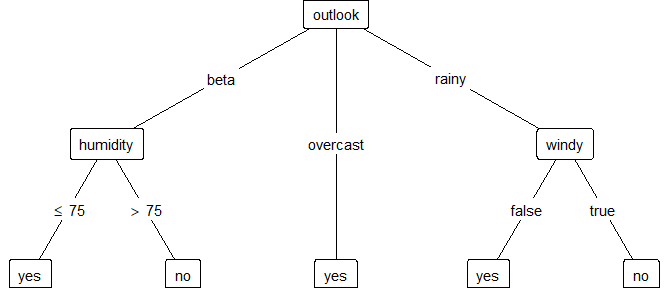As we can see “beta”has not been parsed, even though the argument parse defaults to TRUE and the inequality signs have been parsed. This is due to the fact, that geom_edge_label() detects these signs, generated by get_plot_data() and deparses the rest of the label to prevent unintended parsing. In case we change the default mapping of label this is no longer true. By setting parse to FALSE we can plot the unparsed labels:

ggparty(py) +
geom_edge() +
geom_edge_label(parse = FALSE) +
geom_node_splitvar() +
geom_node_info()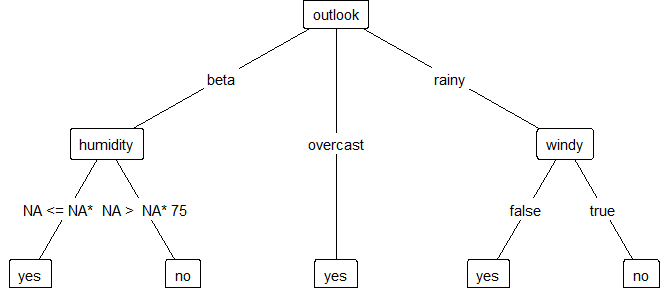On the other hand, if we want to parse the beta which is now one of the splitvariables of outlook, we can set the additional argument parse_all to TRUE.

ggparty(py) +
geom_edge() +
geom_edge_label(parse_all = TRUE) +
geom_node_splitvar() +
geom_node_info()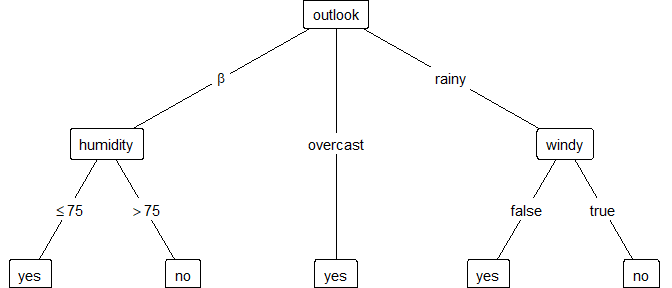## Custom Mapping

If we change the mapping of label, geom_edge_label() will no longer automatically deparse any part of the label. Therefore the argument parse_all has no longer any effect and only parse determines the parsing behaviour.

ggparty(py) +
geom_edge() +
geom_edge_label(mapping = aes(label = paste(breaks_label)),
parse_all = FALSE #has no effect
) +
geom_node_splitvar() +
geom_node_info()Although the specified mapping doesn’t really change anything compared to the default, it makes it harder to prevent “beta” from being parsed, since now nothing gets automatically deparsed.
So if we want to parse certain edges and not others, we need to call geom_edge_label multiple times.

ggparty(py) +
geom_edge() +
geom_edge_label(mapping = aes(label = paste(breaks_label)),
ids = 2,
parse = FALSE
) +
geom_edge_label(mapping = aes(label = paste(breaks_label)),
ids = -2,
parse = TRUE
) +
geom_node_splitvar() +
geom_node_info()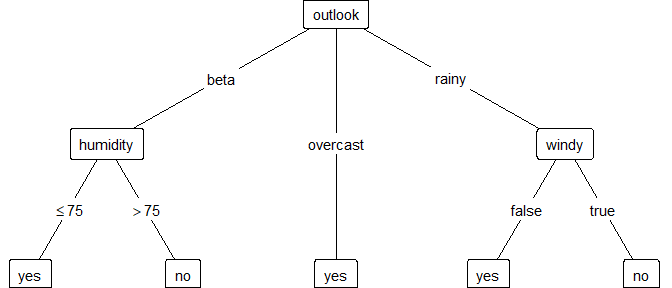These last two plots were just to illustrate the slightly changed mechanics when setting a mapping for label. Let’s now take a look at an example of how to add superscripts to the edge labels. Using the syntax of plotmath we can parse math notations and special characters. So to add a superscript we need to paste a * to tell parse to juxtapose the next symbol which is “NA”. “NA” doesn’t create any character, but is necessary as to add the superscript to it since we can not add it directly to the breaks_label.

ggparty(py) +
geom_edge() +
geom_edge_label(mapping = aes(label = paste(breaks_label, "*NA^", id))) +
geom_node_splitvar() +
geom_node_info()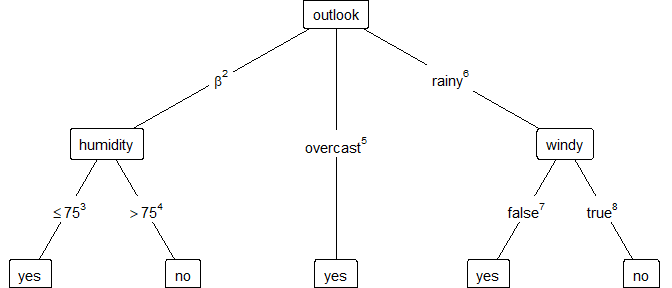If we paste anything that could be parsed but we don’t want it to be, we can deparse it by enclosing it within a pair of \". Remember to add a * at the beginning and the end.

ggparty(py) +
geom_edge() +
geom_edge_label(mapping = aes(label = paste0(breaks_label, "*\"NA^\"*", 1:8))) +
geom_node_splitvar() +
geom_node_info()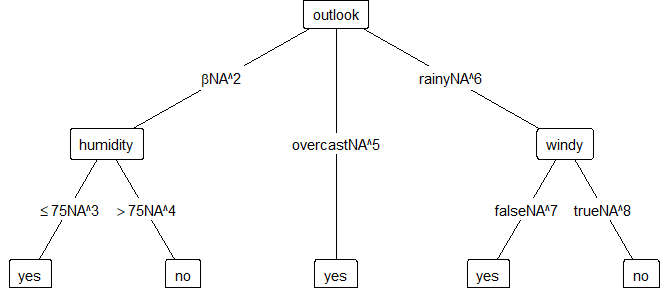# Long breaks_label

In the presence of several levels for some splits we can use the argument splitlevels and plot the levels in several chunks, nudging them slightly in the right position. In some cases the shift argument may also come in handy, as it slides the label along the edge.

library(MASS)
SexTest <- ctree(sex ~ ., data = Aids2)
ggparty(SexTest) +
geom_edge() +
geom_edge_label(splitlevels = 1:2, nudge_y = 0.025) +
geom_edge_label(splitlevels = 3:4, nudge_y = -0.025) +
geom_node_splitvar() +
geom_node_plot(gglist = list(geom_bar(aes(x = "", fill = sex),
position = position_fill())),
shared_axis_labels = TRUE)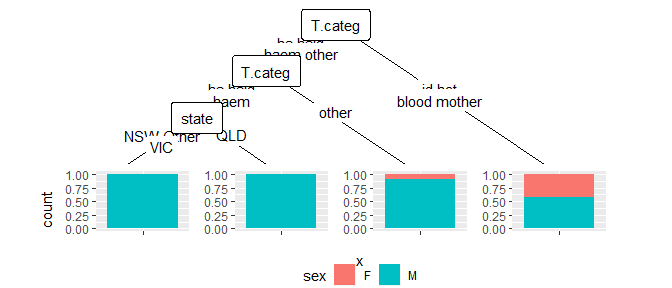Alternatively the argument max_lengthprovides an option to easily truncate the names of the levels.

library(MASS)
SexTest <- ctree(sex ~ ., data = Aids2)
ggparty(SexTest) +
geom_edge() +
geom_edge_label(max_length = 3) +
geom_node_splitvar() +
geom_node_plot(gglist = list(geom_bar(aes(x = "", fill = sex),
position = position_fill())),
shared_axis_labels = TRUE)